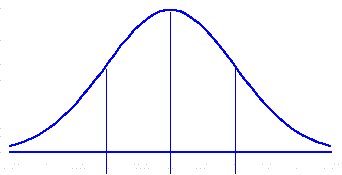Standard Error of Sample Means
 The logic and computational details of this procedure are described in Chapter 9 of Concepts and Applications.

The means of samples of size n, randomly drawn from a normally distributed source population, belong to a normally distributed sampling distribution whose overall mean is equal to the mean of the source population and whose standard deviation ("standard error") is equal to the standard deviation of the source population divided by the square root of n. To calculate the standard error of any particular sampling distribution of sample means, enter the mean and standard deviation (sd) of the source population, along with the value of n, and then click the "Calculate" button.-1sd mean +1sd <== sourcepopulation <== samplingdistribution standard error of sample means = ±
 parameters of source population mean = sd = ± sample size =

 Home Click this link only if you did not arrive here via the VassarStats main page.